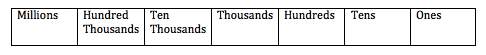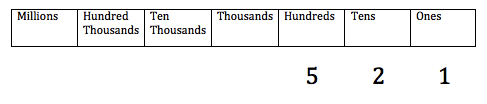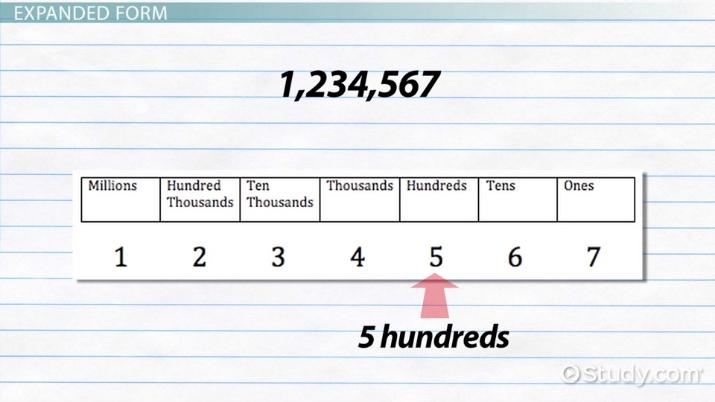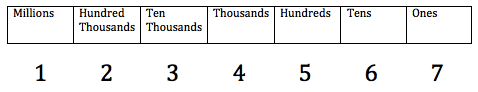# What is Expanded Form in Math? - Definition & Examples

Lesson Transcript
Instructor
Joseph Vigil

Joseph has a master's degree in literature as well as alternative teaching and ESL educator certifications. He has worked with middle school, high school, and college students in writing and language arts.

Expert Contributor
Robert Ferdinand

Robert Ferdinand has taught university-level mathematics, statistics and computer science from freshmen to senior level. Robert has a PhD in Applied Mathematics.

The mathematical process of expanding a number to express the value of each digit/place value is known as expanded form in math. Learn about the definition and process of expanded form and look at some real-world examples. Updated: 09/22/2021

## Place Value

What makes a number mean what it does? Is it simply the digits in the number? For example, let's consider the number 521.

If we switch the digits around, we might get 125. Definitely not the same number. So, it's not just the digits that make a number; it's the digits and their positions within the number. A digit's position in a number and its resulting value is called place value.

The place value chart for our number system looks like this:If we place our original number, 521, into this chart, it now looks like this:Since the digit 5 is in the hundreds place, it means that we have five hundreds, or 500, of whatever we're talking about. Since the two is in the tens place, it means two tens, or 20. And since one is in the ones place, it means one one, or 1.An error occurred trying to load this video.

Try refreshing the page, or contact customer support.

Coming up next: What Is a Number Line?

### You're on a roll. Keep up the good work!

Replay
Your next lesson will play in 10 seconds
• 0:00 Place Value
• 0:55 Expanded Form
• 3:12 Expanded Form to Standard Form
• 3:37 Lesson Summary
Save Save

Want to watch this again later?

Timeline
Autoplay
Autoplay
Speed Speed

## Expanded Form

When we write the number 521, what that number really means is that we have the total of 500 + 20 + 1. We've expanded the number to show the value of each of its digits. When we expand a number to show the value of each digit, we're writing that number in expanded form.

Let's try it with a different, bigger number: 1,234,567. We'll start by placing the number's digits in the place value chart.Now, let's use the chart to determine the value of each digit.

• Since the 1 is in the millions place, it means one million, or 1,000,000
• Since the 2 is in the hundred thousands place, it means two hundred thousands, or 200,000
• Since the 3 is in the ten thousands place, it means three ten thousands, or 30,000
• Since the 4 is in the thousands place, it means four thousands, or 4,000
• Since the 5 is in the hundreds place, it means five hundreds, or 500
• Since the 6 is in the tens place, it means six tens, or 60
• Since the 7 is in the ones place, it means seven ones, or 7

If we write the number out in expanded form, showing the value of each digit, we would write it as 1,000,000 + 200,000 + 30,000 + 4,000 + 500 + 60 + 7. And that's all there is to it!

To unlock this lesson you must be a Study.com Member.

## Practice:What is Expanded Form in Math? - Definition & Examples Quiz

1/5 completed

### In the number 45,902, what is the value of the 5?

Create Your Account To Take This Quiz

As a member, you'll also get unlimited access to over 84,000 lessons in math, English, science, history, and more. Plus, get practice tests, quizzes, and personalized coaching to help you succeed.

It only takes a few minutes to setup and you can cancel any time.

## Practice Questions on Expanded Form

1. Write the following number in expanded form: 23,465.

2. Write in expanded form: 5,065.

3. Convert to standard form: 40000 + 5000 + 700 + 60 + 8.

4. Convert to standard form: 8000 + 700 + 5.

1. Considering the place value of each digit with 2 being in the ten-thousands place, 3 in the thousands place, 4 in the hundreds place, 6 in the tens place and 5 in the ones place, we get

23,465 = 20,000 + 3,000 + 400 + 60 + 5 as the expanded form.

2. Considering the place value of each digit with 5 in the thousands place, 0 in the hundreds place, 6 in the tens place, and 5 in the ones place, we get 5,065 = 5,000 + 60 + 5 as the expanded form.

3. To convert to standard form, we simply look at the place values of the numbers and add them to obtain 40,000 + 5,000 + 700 + 60 + 8 = 45,768 as the standard form of the number. Really we just need to line up the numbers starting with the highest order down to the lowest order.

4. To convert to standard form, we simply look at the place values of the numbers and add them to obtain 8,000 + 700 + 5 = 8,705 as the standard form of the number. Really we just need to line up the numbers starting with the highest order down to the lowest order. Notice there is a zero in the tens place as there is no tens term in the expanded form.

### Register to view this lesson

Are you a student or a teacher?